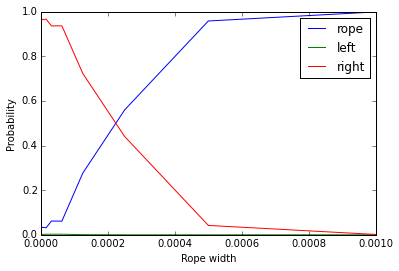# The importance of the region of practical equivalence (ROPE)

The difference between two classifiers (algorithms) can be very small; however there are no two classifiers whose
accuracies are perfectly equivalent
.

By using an null hypothesis significance test (NHST), the null hypothesis is that the classifiers are equal. However, the null hypothesis is practically always false!
By rejecting the null hypothesis NHST indicates that the null hypothesis is unlikely; but this is known even before running the experiment.

We will use the Python modules `signtest` and `signrank` in `bayesiantests` (see our GitHub repository).

#### Bayesian tests can assess when two classifiers are practically equivalent

Can we say anything about the probability that two classifiers are practically equivalent (e.g., j48 is practically equivalent to j48gr)?
NHST cannot answer this question, while Bayesian analysis can.

We need to define the meaning of practically equivalent.

#### How to define a rope?

The rope depends:

1. on the metric we use for comapring classifiers (accuracy, logloss etc.);
2. on our subjective definition of practical equivalence (domain specific).

Accuracy is a number in \$[0,1]\$. For practical applications, it is sensible to define that two classifiers whose mean difference of accuracies is less that \$1\%\$ (\$0.01\$) are practically equivalent.
A difference of accuracy of \$1\%\$ is neglegible in practice.

The interval \$[-0.01,0.01]\$ can thus be used to define a region of practical equivalence for classifiers.

See it in action.

We load the classification accuracies of J48 and J48gr on 54 UCI datasets from the file `Data/accuracy_j48_j48gr.csv`. For simplicity, we will skip the header row and the column with data set names.

In :
```import numpy as np
scores = np.loadtxt('Data/accuracy_j48_j48gr.csv', delimiter=',', skiprows=1, usecols=(1, 2))
names = ("J48", "J48gr")
```

#### Bayesian sign test

Function `signtest(x, rope, prior_strength=1, prior_place=ROPE, nsamples=50000, verbose=False, names=('C1', 'C2'))` computes the Bayesian signed-rank test and returns the probabilities that the difference (the score of the first classifier minus the score of the first) is negative, within rope or positive.

In :
```import bayesiantests as bt
left, within, right = bt.signtest(scores, rope=0.01,verbose=True,names=names)
```
```P(J48 > J48gr) = 0.0, P(rope) = 1.0, P(J48gr > J48) = 0.0
```

The first value (`P(J48 > J48gr)`) is the probability that the first classifier (the left column of `x`) has a higher score than the second (or that the differences are negative, if `x` is given as a vector).

The second value (`P(rope)`) is the probability that they are practically equivalent.

The third value (`P(J48gr > J48)`) is equal to `1-P(J48 > J48gr)-P(rope)`.

The probability of the rope is equal to \$1\$ and, therefore, we can say that they are equivalent (for the given rope).

#### Zoom in

Decision tree grafting (J48gr) was developed to demonstrate that a preference for less complex trees (J48) does not serve to improve accuracy. The point is that J48gr has a consistent (albeit small) improvements in accuracy than J48.

The advantage of having a rope is that we can test this hypothesis from a statistical point of view.

#### Is the difference more than 0.001 (1/1000)?

In :
```left, within, right = bt.signtest(scores, rope=0.001,verbose=True,names=names)
```
```P(J48 > J48gr) = 0.0, P(rope) = 0.99822, P(J48gr > J48) = 0.00178
```

No the difference is less than 0.001 with probability 0.99

#### Is the difference more than 0.0001 (1/10000)?

In :
```left, within, right = bt.signtest(scores, rope=0.0001,verbose=True,names=names)
```
```P(J48 > J48gr) = 0.00164, P(rope) = 0.14482, P(J48gr > J48) = 0.85354
```

The difference is therefore in the order of 0.0001. The difference is very small (around 1/10000), but in favour of J48gr.

#### Is it due to the prior?

In :
```left, within, right = bt.signtest(scores, rope=0.0001,prior_place=bt.RIGHT,verbose=True,names=names)
```
```P(J48 > J48gr) = 0.00118, P(rope) = 0.0868, P(J48gr > J48) = 0.91202
```

The conclusions are in this case sensitive to the prior (posterior changes 0.05 points). However, the overall conclusion does not change much. The difference is very small (around 1/10000), but in favour of J48gr.

#### Let us plot them

We can plot the three probabilities as function of the Rope width.

In :
```%matplotlib inline
import matplotlib.pyplot as plt
left=np.zeros((10,1))
within=np.zeros((10,1))
right=np.zeros((10,1))
for i in range(9,-1,-1):
left[i], within[i], right[i] = bt.signtest(scores, rope=0.001/2**i,names=names)
plt.plot(0.001/(2**np.arange(0,10,1)),within)
plt.plot(0.001/(2**np.arange(0,10,1)),left)
plt.plot(0.001/(2**np.arange(0,10,1)),right)
plt.legend(('rope','left','right'))
plt.xlabel('Rope width')
plt.ylabel('Probability')
```
Out:
`<matplotlib.text.Text at 0x7fb81a5fc0b8>`#### Using Signrank test

We can also use the signrank that is more sensitivie to differences.

In :
```left, within, right = bt.signrank(scores, rope=0.001,verbose=True,names=names)
```
```P(J48 > J48gr) = 0.0, P(rope) = 0.82288, P(J48gr > J48) = 0.17712
```
In :
```left, within, right = bt.signrank(scores, rope=0.0001,verbose=True,names=names)
```
```P(J48 > J48gr) = 6e-05, P(rope) = 0.0007, P(J48gr > J48) = 0.99924
```

However, the conclusion is very similar. The difference is very small (1/10000), but in favour of J48gr.

In [ ]:
```
```

This site uses Akismet to reduce spam. Learn how your comment data is processed.